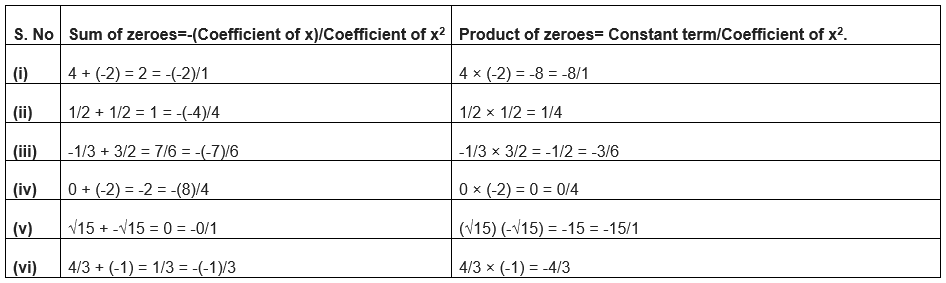# NCERT book Solutions for Class 10 Maths Polynomials Exercise 2.2

## NCERT book Solutions for Class 10 Maths Chapter 2 Exercise 2.2

In this page we have NCERT book Solutions for Class 10th Maths:Polynomials for Exercise 2.2 on page 33 . Hope you like them and do not forget to like , social_share and comment at the end of the page.

Question 1
Find the zeroes of the following quadratic polynomials and verify the relationship between the zeroes and the coefficients.
(i) x2 – 2x – 8
(ii) 4s2 – 4s + 1
(iii) 6x2 – 3 – 7x
(iv) 4u2 + 8u
(v)t2 – 15
(vi) 3x2 – x – 4
(i) x2 – 2x – 8
= x2 -4x+ 2x – 8
= (x - 4) (x + 2)
Therefore, the zeroes of x2 – 2x – 8 are 4 and -2.
(ii) 4s2 – 4s + 1
From (a-b)2 = a2 -2ab + b2
= (2s-1)2
Therefore, the zeroes of 4s2 - 4s + 1 are 1/2 and 1/2.
(iii) 6x2 – 3 – 7x
= 6x– 7x – 3
= 6x2 -9x +2x -3
= (3x + 1) (2x - 3)
Therefore, the zeroes of 6x2 - 3 - 7x are -1/3 and 3/2.
(iv) 4u2 + 8u
= 4u2 + 8u
= 4u(u + 2)
Therefore, the zeroes of 4u2 + 8u are 0 and - 2.
(v) t2 – 15
From (a2 -b2) =(a-b) (a+b)
= (t - √15) (t + √15)
Therefore, the zeroes of t2 - 15 are √15 and -√15.
(vi) 3x2 – x – 4
=3x2 – 4x+3x – 4
= (3x - 4) (x + 1)
Therefore, the zeroes of 3x2 – x – 4 are 4/3 and -1.
Verification of the relationship between the zeroesQuestion 2
Find a quadratic polynomial each with the given numbers as the sum and product of its zeroes respectively.
(i) 1/4, -1
(ii) √2, 1/3
(iii) 0, √5
(iv) 1,1
(v) -1/4 ,1/4
(vi) 4,1

(i) 1/4 , -1 Let the polynomial be ax2 + bx + c, and its zeroes be p and q
p + q = 1/4 = -b/a
pq = -1 = -4/4 = c/a
Now we have two method to find the quadratic polynomial
Method -1
The polynomial can be written as
k[x2 - (sum of roots)x + (products of roots)]
So,
$k[x^2 - (\frac {1}{4})x -1]$
Taking k=4
$4x^2 -x -4$
Method -2
If we take a= 4, then b = -1, c= -4

Therefore, the quadratic polynomial is 4x2 - x -4.
We could choose any of the method to get the polynomial

(ii) √2 , 1/3
Let the polynomial be ax2 + bx + c, and its zeroes be p and q
p + q = √2 = 3√2/3 = -b/a
pq = 1/3 = c/a
If a = 3, then b = -3√2, c = 1
Therefore, the quadratic polynomial is 3x2 -3√2x +1.

(iii) 0, √5
Let the polynomial be ax2 + bx + c, and its zeroes be p and q
p + q = 0 = 0/1 = -b/a
pq = √5 = √5/1 = c/a
If a = 1, then b = 0, c = √5
Therefore, the quadratic polynomial is x2 + √5.

(iv) 1, 1
Let the polynomial be ax2 + bx + c, and its zeroes be p and q
p + q = 1 = 1/1 = -b/a
pq = 1 = 1/1 = c/a
If a = 1, then b = -1, c = 1
Therefore, the quadratic polynomial is x2 - x +1.

(v) -1/4 ,1/4
Let the polynomial be ax2 + bx + c, and its zeroes be p and q
p + q = -1/4 = -b/a
pq = 1/4 = c/a
If a = 4, then b = 1, c = 1
Therefore, the quadratic polynomial is 4x2 + x +1.

(vi) 4,1
Let the polynomial be ax2 + bx + c, and its zeroes be p and q
p + q = 4 = 4/1 = -b/a
pq = 1 = 1/1 = c/a
If a = 1, then b = -4, c = 1
Therefore, the quadratic polynomial is x2 - 4x +1.

## Summary

1. NCERT book Solutions for Class 10th Maths:Polynomials Exercise 2.2 has been prepared by Expert with utmost care. If you find any mistake.Please do provide feedback on mail. You can download the solutions as PDF in the below Link also
2. This chapter 2 has total 4 Exercise 2.1 ,2.2,2.3 and 2.4. This is the Second exercise in the chapter.You can explore previous exercise of this chapter by clicking the link belowGo back to Class 10 Main Page using below links

### Practice Question

Question 1 What is $1 - \sqrt {3}$ ?
A) Non terminating repeating
B) Non terminating non repeating
C) Terminating
D) None of the above
Question 2 The volume of the largest right circular cone that can be cut out from a cube of edge 4.2 cm is?
A) 19.4 cm3
B) 12 cm3
C) 78.6 cm3
D) 58.2 cm3
Question 3 The sum of the first three terms of an AP is 33. If the product of the first and the third term exceeds the second term by 29, the AP is ?
A) 2 ,21,11
B) 1,10,19
C) -1 ,8,17
D) 2 ,11,20